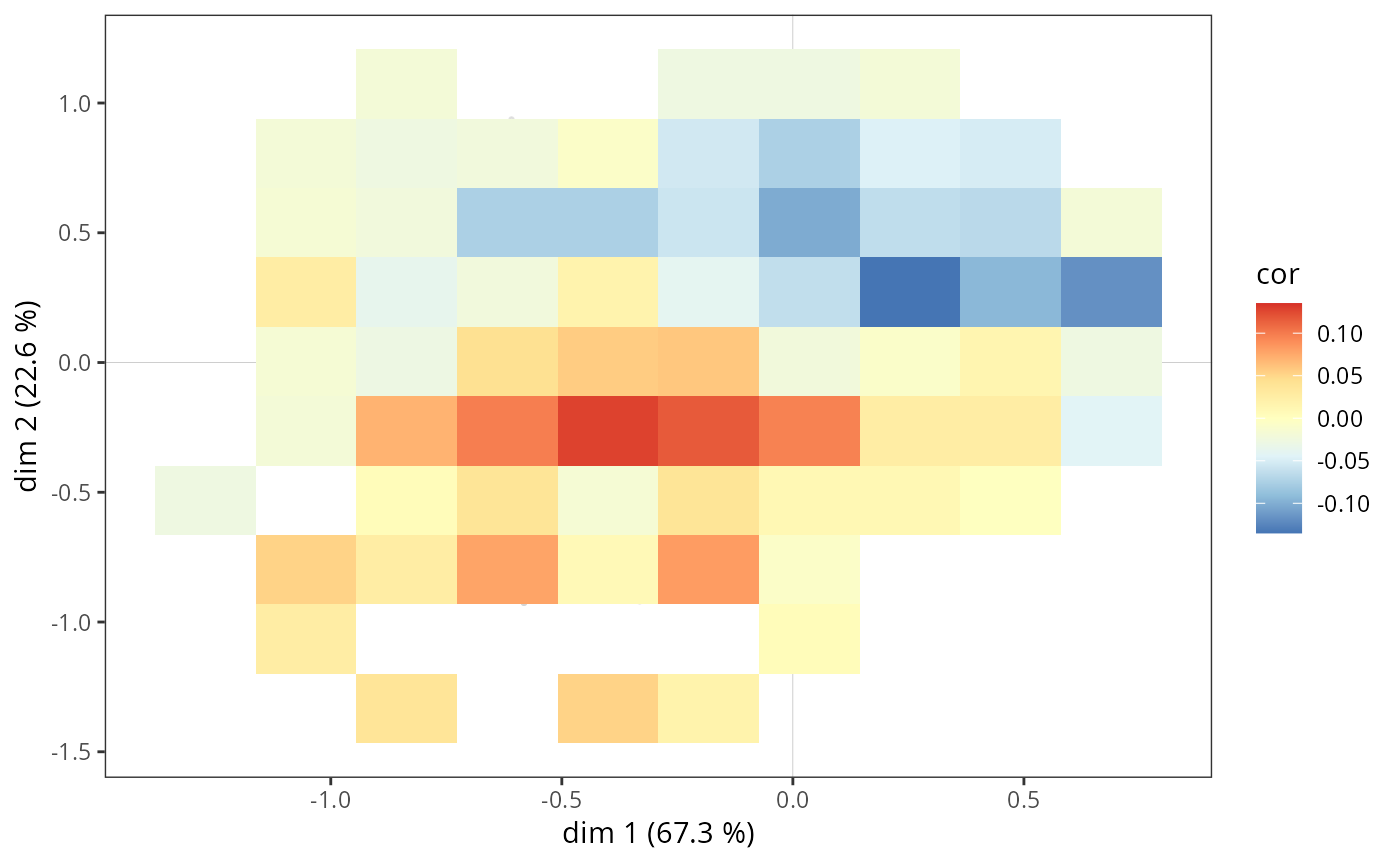Adds a heatmap representing the correlation coefficients to a MCA cloud of individuals, for a numerical supplementary variable or one category of a categorical supplementary variable.

ggadd_corr(p, resmca, var, cat = levels(var), axes = c(1,2),
xbins = 20, ybins = 20, min.n = 1, pal = "RdYlBu", limits = NULL, legend = "right")

## Arguments

p

ggplot2 object with the cloud of variables

resmca

object of class MCA, speMCA, csMCA, stMCA or multiMCA

var

factor or numerical vector. The supplementary variable used for the heatmap.

cat

character string. The category of var to plot (by default, the first level of var is plotted). Only used if var is a factor.

axes

numeric vector of length 2, specifying the components (axes) to plot. Default is c(1,2).

xbins

integer. Number of bins in the x axis. Default is 20.

ybins

integer. Number of bins in the y axis. Default is 20.

min.n

integer. Minimal number of points for a tile to be drawn. By default, every tiles are drawn.

pal

character string. Name of a (preferably diverging) palette from the RColorBrewer package. Default is "RdYlBu".

limits

numerical vector of length 2. Lower and upper limits of the correlation coefficients for the color scale. Should be centered around 0 for a better view of under/over-representations (for example c(-0.2,0.2)). By default, the maximal absolute value of the correlation coefficients is used.

legend

the position of legends ("none", "left", "right", "bottom", "top", or two-element numeric vector). Default is right.

## Value

a ggplot2 object

## Details

For each tile of the heatmap, a correlation coefficient is computed between the supplementary variable and the fact of belonging to the tile. This gives a view of the under/over-representation of the supplementary variable according to the position in the cloud of individuals.

## Author

Nicolas Robette

ggcloud_variables, ggadd_supvar, ggadd_supvars, ggadd_kellipses, ggadd_ellipses, ggadd_interaction, ggsmoothed_supvar, ggadd_chulls, ggadd_density

## Examples

# specific MCA of Taste example data set
data(Taste)
junk <- c("FrenchPop.NA", "Rap.NA", "Rock.NA", "Jazz.NA", "Classical.NA",
"Comedy.NA", "Crime.NA", "Animation.NA", "SciFi.NA", "Love.NA",
"Musical.NA")
mca <- speMCA(Taste[,1:11], excl = junk)
# correlation heatmap for Age = 50+
p <- ggcloud_indiv(mca, col = "lightgrey")
ggadd_corr(p, mca, var = Taste\$Age, cat = "50+", xbins = 10, ybins = 10)